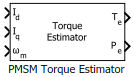# PMSM Torque Estimator

Estimate electromechanical torque and power

• Library:
• Motor Control Blockset / Controls / Control Reference

•## Description

The PMSM Torque Estimator block generates electromechanical torque and power estimates to enable field-oriented control of Permanent Magnet Synchronous Motor (PMSM).

The block accepts feedback values of d- and q-axis current and mechanical speed as inputs.

### Equations

If you select `Per-Unit (PU)` in the Input units parameter, the block converts the inputs to SI units before performing any computation. After calculating the output, the block converts the output back to per unit values.

The following equations describe the computation of electromechanical torque and power estimates by the block.

`${T}_{e}=\frac{3}{2}p\left\{{\lambda }_{m}{I}_{q}+\left({L}_{d}-{L}_{q}\right){I}_{d}{I}_{q}\right\}$`
`${P}_{\text{e}}={T}_{e}×{\omega }_{\text{m}}$`

where:

• ${L}_{\text{d}}$ and ${L}_{\text{q}}$ are the d-axis and q-axis stator winding inductances (Henry).

• ${I}_{\text{d}}$ and ${I}_{\text{q}}$ are the d-axis and q-axis current (Amperes).

• ${\lambda }_{\text{m}}$ is the permanent magnet flux linkage (Weber).

• $p$ is the number of pole pairs available in the motor.

• ${\omega }_{m}$ is the mechanical speed of the rotor (Radians/ sec).

## Ports

### Input

expand all

Current along the d-axis of the rotating dq reference frame.

Data Types: `single` | `double` | `fixed point`

Current along the q-axis of the rotating dq reference frame.

Data Types: `single` | `double` | `fixed point`

Mechanical speed of the rotor.

Data Types: `single` | `double` | `fixed point`

### Output

expand all

Electromechanical torque of the rotor.

Data Types: `single` | `double` | `fixed point`

Electromechanical power of the rotor.

Data Types: `single` | `double` | `fixed point`

## Parameters

expand all

Number of pole pairs available in the motor.

Stator winding inductance (henry) along the direct-axis of the rotating dq reference frame.

Stator winding inductance (henry) along the quadrature-axis of the rotating dq reference frame.

Peak permanent magnet flux linkage (weber).

Unit of the input values.

Nominal voltage (in Volts) that corresponds to one per unit voltage value.

#### Dependencies

To enable this parameter, set Input units to `Per-Unit (PU)`.

Nominal current (in Amperes) that corresponds to one per unit current value.

#### Dependencies

To enable this parameter, set Input units to `Per-Unit (PU)`.

Nominal speed (in rpm) that corresponds to one per unit speed value.

#### Dependencies

To enable this parameter, set Input units to `Per-Unit (PU)`.

Get ebook